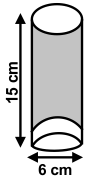﻿ Maths Olympiad (CMO) Sample Paper & Questions for Class 10

# CREST Mathematics Olympiad Class 10 Sample Papers

Sample PDF of CREST Mathematics Olympiad for Class 10:

## Syllabus:

Section 1: Real Numbers, Polynomials, Pair of Linear Equations in Two Variables, Quadratic Equations, Arithmetic Progressions, Triangles, Coordinate Geometry, Introduction to Trigonometry, Some Applications of Trigonometry, Circles, Constructions, Areas Related to Circles, Surface Areas and Volumes, Statistics, Probability.

Achievers Section: Higher Order Thinking Questions - Syllabus as per Section 1

 Q.1 If the first, second and the last terms of an AP are a, b, c, respectively, then the sum is:
 Q.2 A three digits number was chosen at random. Find the probability that its hundred's digit, ten's digit and unit's digit are consecutive integers in descending order:
 Q.3 If [(x - a)/(b + c)] + [(x - b)/(c + a)] + [(x - c)/(a + b)] = 3, then find the value of x:
 Q.4 Two verticles of a triangle are (5, -1) and (-2, 3). If the orthocentre of the triangle is the origin, find the third vertex:
 Q.5 In the adjoining figure, the bottom of the glass has a hemispherical raised portion. If the glass is filled with orange juice, then find the quantity of juice which a person will get:Q.6 Which among the following is a singleton set?
 Q.7 The angles of elevation of the top of a tower from two points at distances m and n metres are complementary. If the two points and the base of the tower are on the same straight line, then what will be the height of the tower?
 Q.8 If the sum of the roots of the equation ax2 + bx + c = 0 is equal to the sum of their squares, then which one of the following is correct?
 Q.9 If p1 and p2 are two odd prime numbers such that p1 > p2, then p12 - p22 is:
 Q.10 In the binomial expansion of (a - b)n, n ≥ 5 the sum of the 5th and 6th terms is zero. Find the value of a/b: